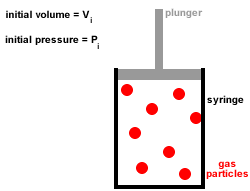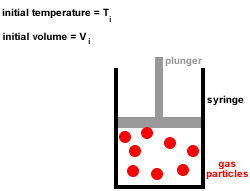Kinetic Theory of Gases Tutorial

Key Concepts

• The kinetic theory, or the kinetic-molecular theory, of gases explains the properties of ideal gases.
• The kinetic theory of gases is based on the following four assumptions:

1. A gas is composed of a large number of tiny particles which are so small that their sizes are negligible compared to the average distances between them, that is, most of the volume of a gas is just empty space.

2. Gas particles are in constant, random, straight-line motion, colliding with each other and the walls of the container.
All collisions are elastic, there is no net loss or gain of kinetic energy at each collision.

3. Gas particles move independently of each other, there are no forces of attraction or repulsion between them.

4. At any given instant there is a wide range of particle speeds and therefore a wide range of molecular kinetic energies, however, the average kinetic energy of all the gas particles is proportional to the absolute temperature (temperature in kelvin).

The kinetic energy, Ek or K.E, of a single gas particle is given by the equation below:

Ek = ½mv2

where m = mass of gas particle and v = speed of gas particle

• Gases will deviate from ideal gas behaviour if one or more of these assumptions does not apply

No ads = no money for us = no free stuff for you!

Kinetic Theory Applications: Pressure-Volume Relationships at Constant Temperature (Boyle's Law)

Pressure (force per unit area) is due to gas particles colliding with the walls of the container.

At constant temperature, the average kinetic energy remains constant, so the average speed of the gas particles is constant.

Reducing the volume available to the gas reduces the distances between gas particles which increases the number of collisions between gas particles and the walls of the container so the pressure inside the container increases.Increasing the volume available to the gas increases the distance between gas particles which reduces the number of collisions between gas particles and the walls of the container so the pressure inside the container decreases.

At high pressures the interactions between particles become significant and the gas will no longer behave like an ideal gas.

Do you know this?

Play the game now!

Kinetic Theory Applications: Pressure-Temperature Relationships at Constant Volume

As the temperature increases, the kinetic energy and the average speed of the gas particles increases.

At constant volume this results in more collisions between the gas particles and the walls of the container, so the pressure inside the container increases.

At temperatures approaching absolute zero (0 Kelvin), the motion of the particles becomes so slow that interactions between the particles become significant and the gas no longer behaves like an ideal gas.

Do you understand this?

Take the test now!

Kinetic Theory Applications: Volume-Temperature Relationships at Constant Pressure (Charles' Law)

As the temperature increases, the average kinetic energy and speed of the gas particles increases.

In order to maintain a constant pressure, the volume of the container must increase, increasing the distance between gas particles and the walls of the container.Reducing the temperature reduces the average kinetic energy and speed of the gas particles.
In order to maintain constant pressure, the volume of the container must decrease, reducing the distance between gas particles.

Can you apply this?

Join AUS-e-TUTE!

Take the exam now!

Kinetic Theory Applications: Mole-Pressure Relationships at Constant Temperature (Dalton's Law of Partial Pressures)

At constant temperature, the gas pressure is related to the number of gas particles colliding with the walls of the container.

Adding more gas particles, as long as those gases do not chemically react with each other, will increase the gas pressure.

If 25% of the gas particles in a mixture are gas a, and 75% are gas b, then 25% of the total gas pressure is due to gas a, and 75% of the total gas pressure is due to gas b.

Can you apply this?

Join AUS-e-TUTE!

Take the exam now!